# Calculus 3 : Directional Derivatives

## Example Questions

← Previous 1 3 4 5 6 7 8 9 35 36

### Example Question #1 : Directional Derivatives

Calculate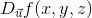, where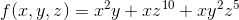in the direction of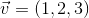.

Possible Answers: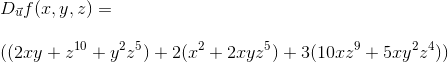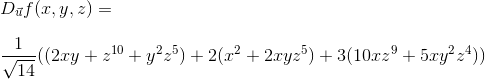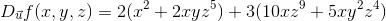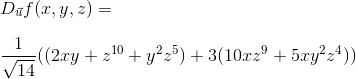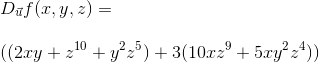Correct answer:Explanation:

The first thing to check is to see if the direction vector is a unit vector.

In order to see if it is a unit vector, we need to take the magnitude and see if it is equal to.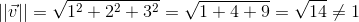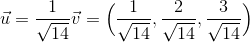Now we are going to take partial derivatives in respect to, and then, and then multiply each partial by the component of the unit vector that corresponds to it.

The formula is: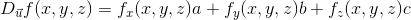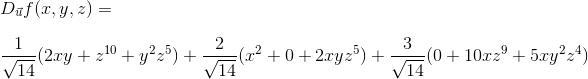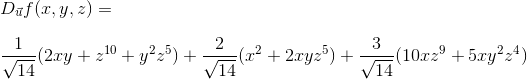### Example Question #2 : Directional Derivatives

Calculate, where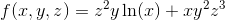in the direction of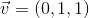.

Possible Answers: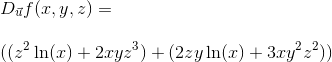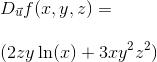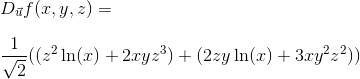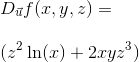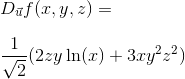Correct answer:Explanation:

The first thing to check is to see if the direction vector is a unit vector.

In order to see if it is a unit vector, we need to take the magnitude and see if it is equal to.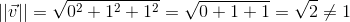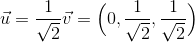Now we are going to take partial derivatives in respect to, and then, and then multiply each partial by the component of the unit vector that corresponds to it.

The formula is: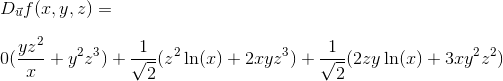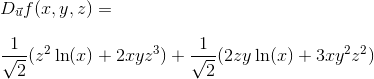### Example Question #3 : Directional Derivatives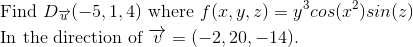Possible Answers: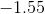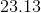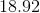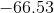Correct answer:Explanation: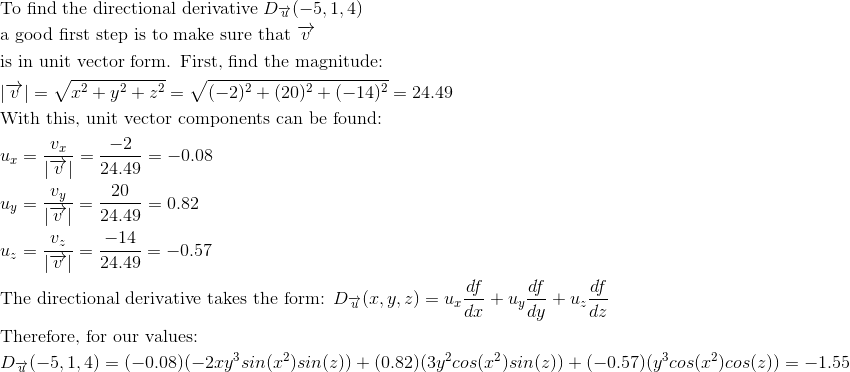### Example Question #4 : Directional Derivatives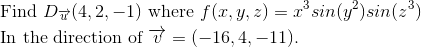Possible Answers: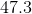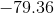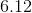Correct answer:Explanation: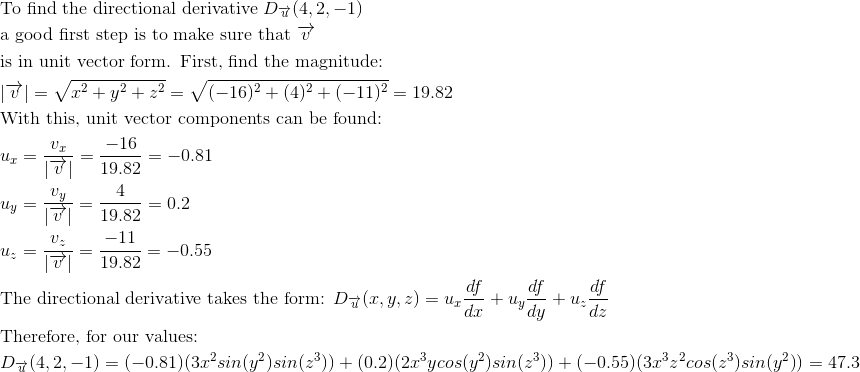### Example Question #5 : Directional Derivatives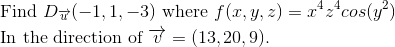Possible Answers: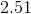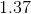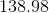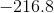Correct answer:Explanation: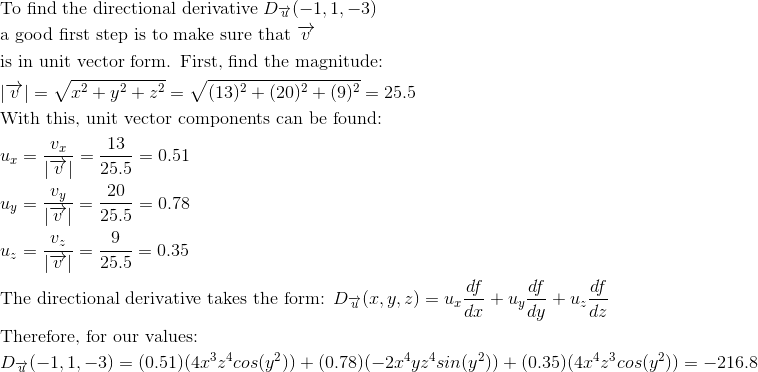### Example Question #6 : Directional Derivatives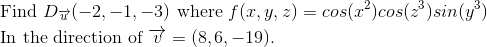Possible Answers: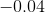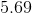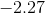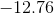Correct answer:Explanation: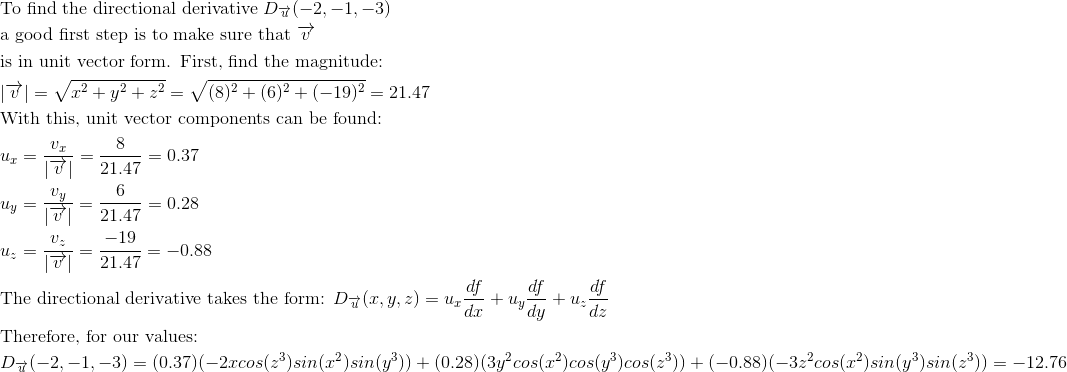### Example Question #7 : Directional Derivatives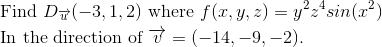Possible Answers: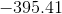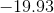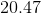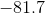Correct answer:Explanation: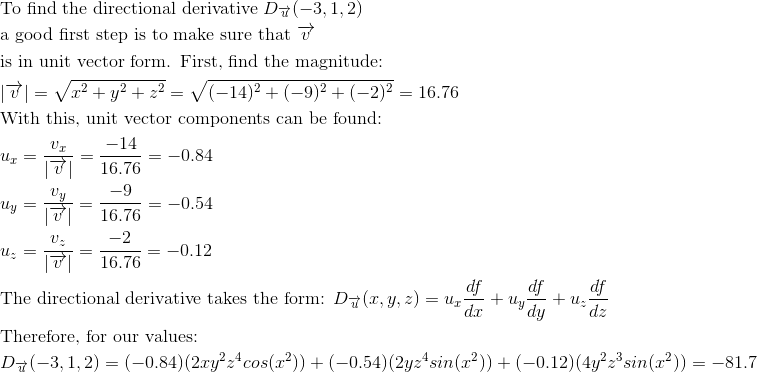### Example Question #8 : Directional Derivatives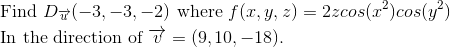Possible Answers: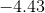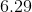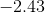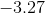Correct answer:Explanation: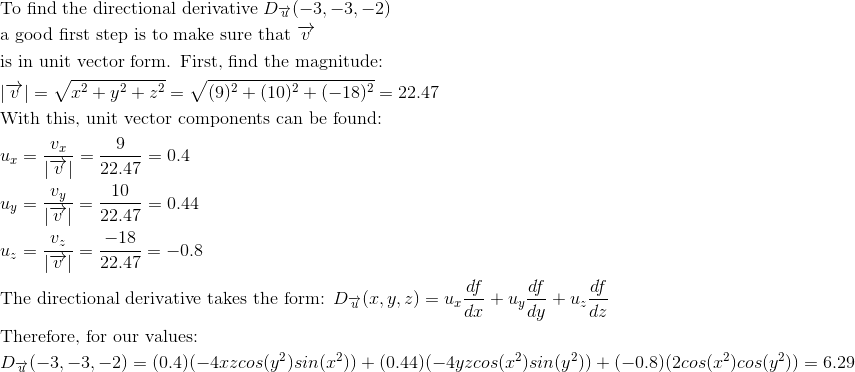### Example Question #9 : Directional Derivatives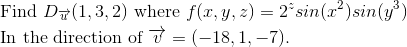Possible Answers: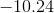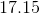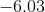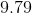Correct answer:Explanation: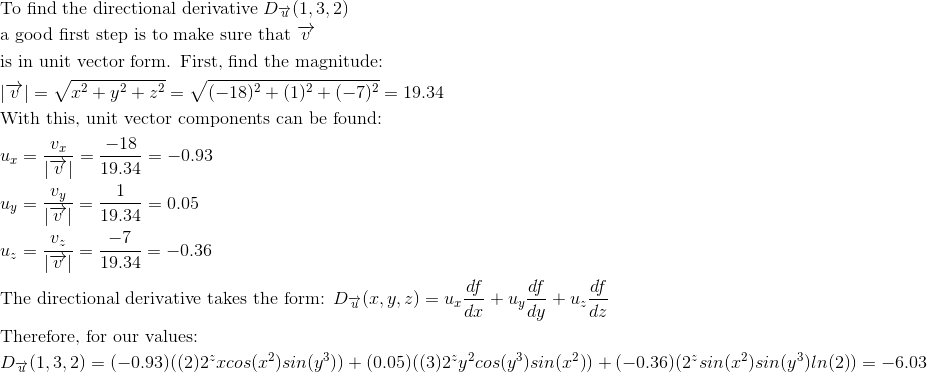### Example Question #10 : Directional Derivatives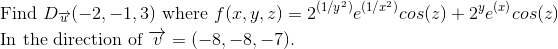Possible Answers: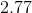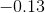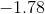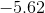Correct answer:Explanation: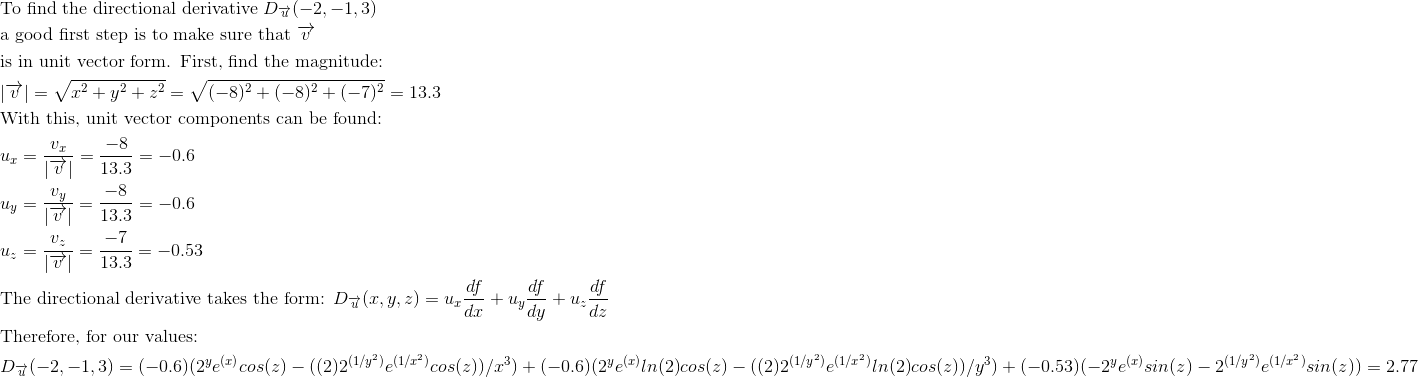← Previous 1 3 4 5 6 7 8 9 35 36

### All Calculus 3 Resources